# Constant Part Constant Total Constant Difference All items

• Slides: 15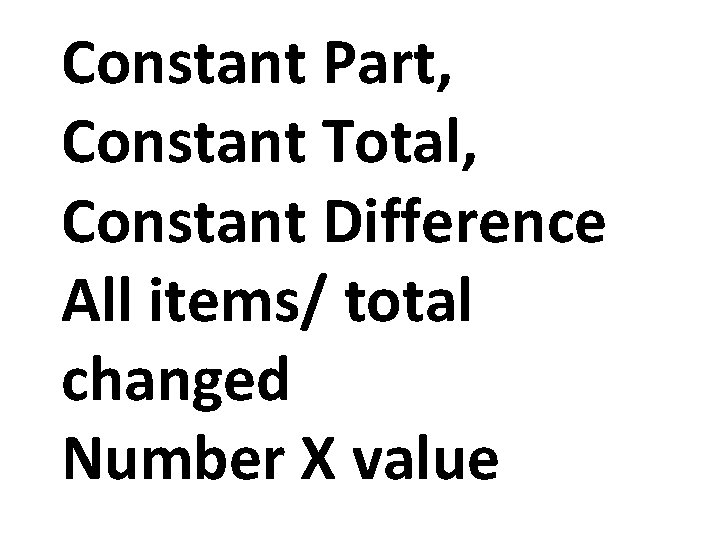Constant Part, Constant Total, Constant Difference All items/ total changed Number X value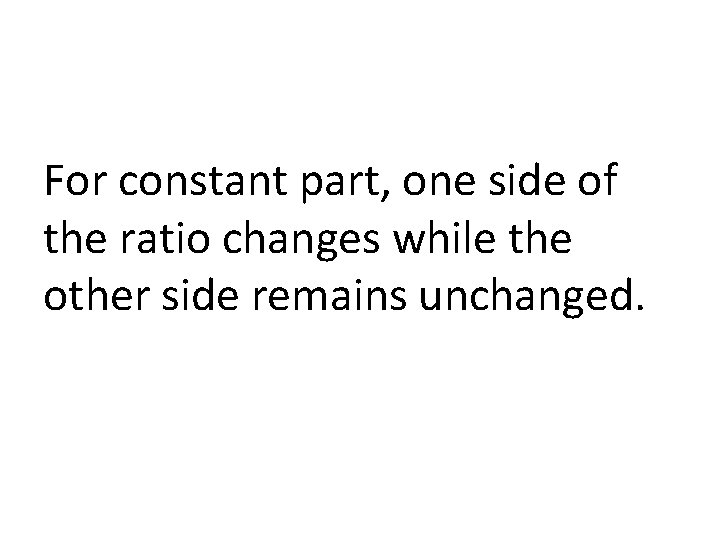For constant part, one side of the ratio changes while the other side remains unchanged.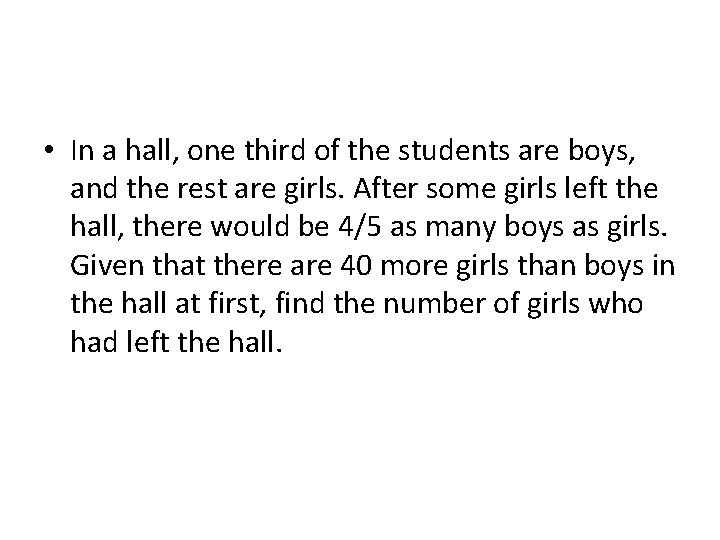• In a hall, one third of the students are boys, and the rest are girls. After some girls left the hall, there would be 4/5 as many boys as girls. Given that there are 40 more girls than boys in the hall at first, find the number of girls who had left the hall.External Transfer (unchanged quantity) Mrs Lim baked thrice as many cakes as Mrs Loh. After Mrs Lim gave away 115 cakes, Mrs Loh had twice as many cakes as her. How many cakes did Mrs Lim have left?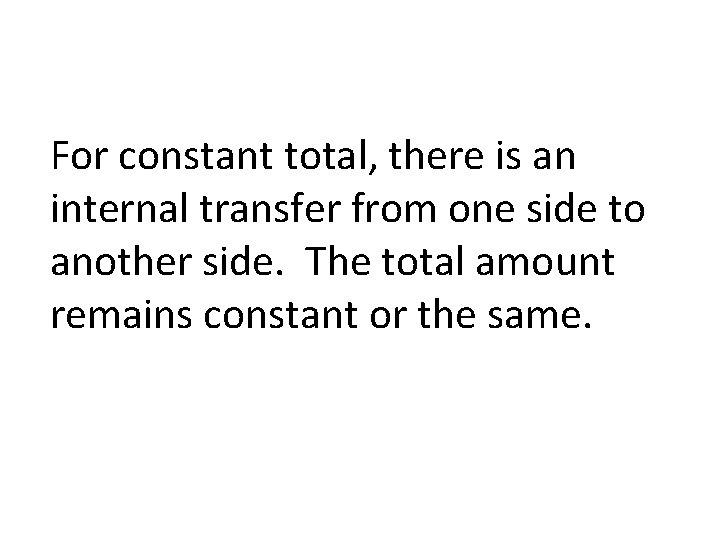For constant total, there is an internal transfer from one side to another side. The total amount remains constant or the same.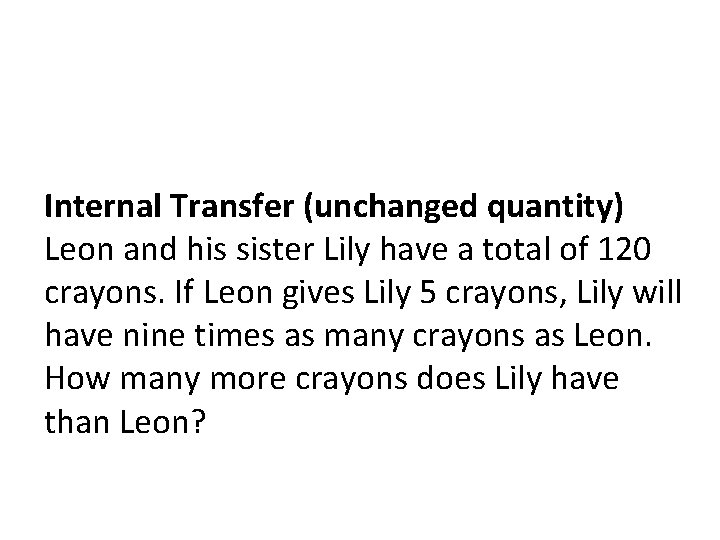Internal Transfer (unchanged quantity) Leon and his sister Lily have a total of 120 crayons. If Leon gives Lily 5 crayons, Lily will have nine times as many crayons as Leon. How many more crayons does Lily have than Leon?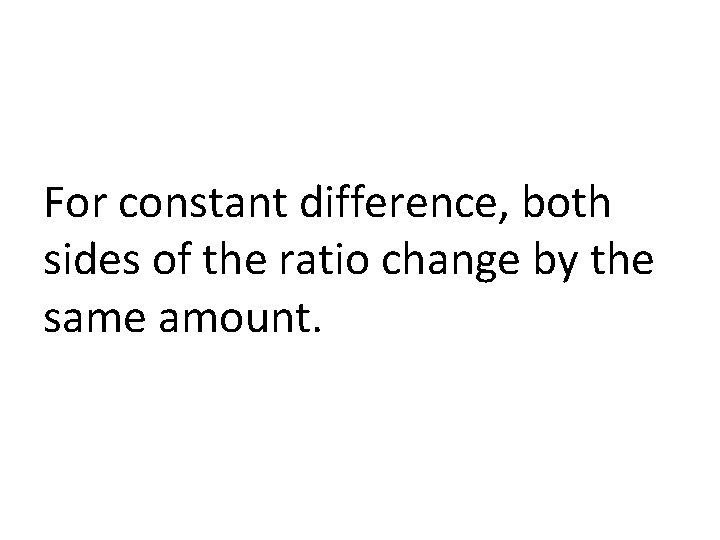For constant difference, both sides of the ratio change by the same amount.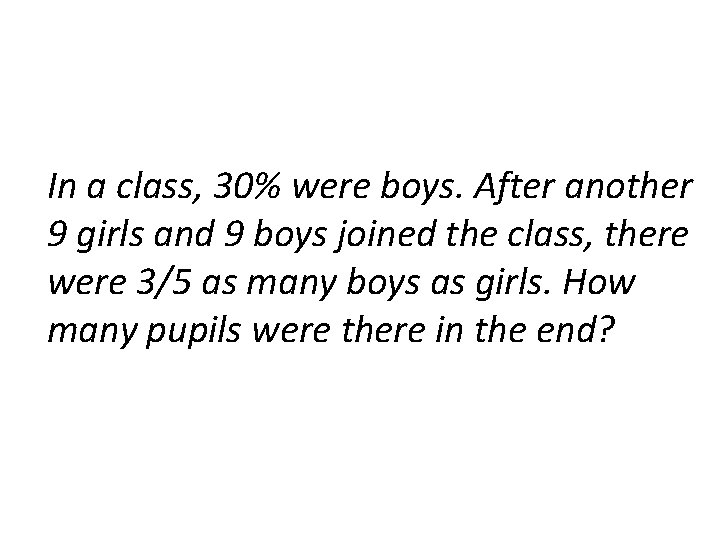In a class, 30% were boys. After another 9 girls and 9 boys joined the class, there were 3/5 as many boys as girls. How many pupils were there in the end?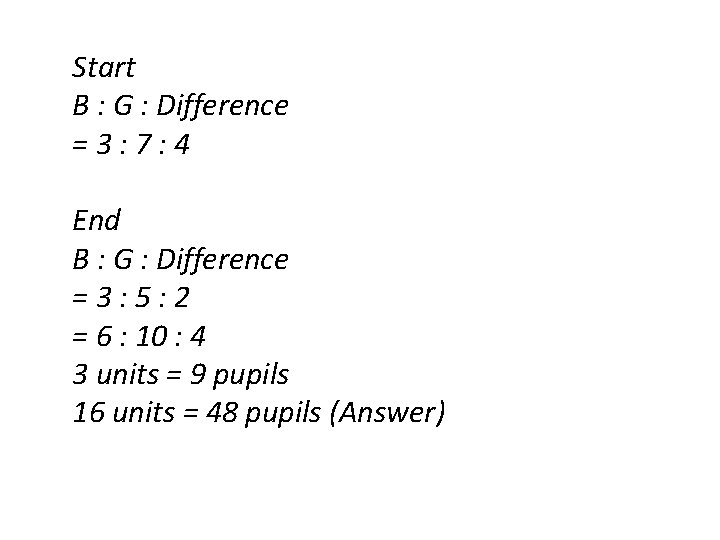Start B : G : Difference = 3 : 7 : 4 End B : G : Difference = 3 : 5 : 2 = 6 : 10 : 4 3 units = 9 pupils 16 units = 48 pupils (Answer)All items / total changed Finally, both sides of the ratio change and total changes.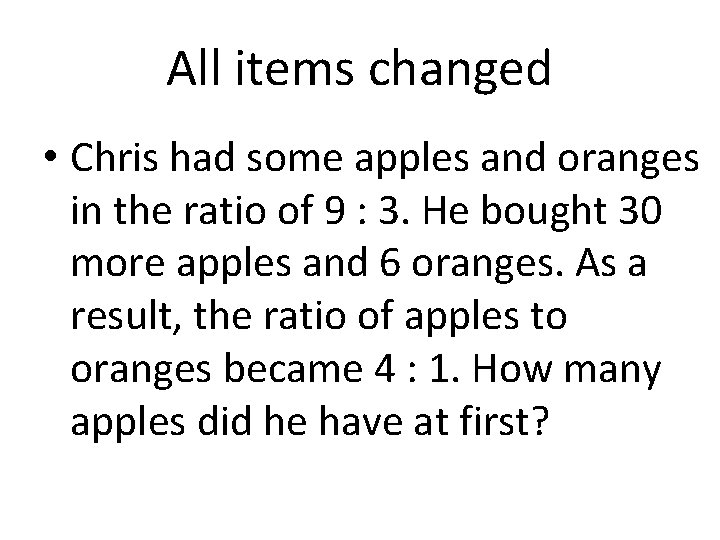All items changed • Chris had some apples and oranges in the ratio of 9 : 3. He bought 30 more apples and 6 oranges. As a result, the ratio of apples to oranges became 4 : 1. How many apples did he have at first?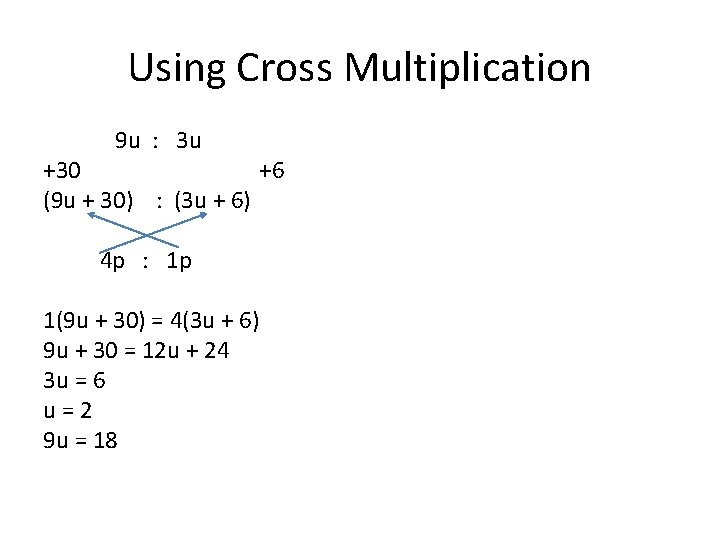Using Cross Multiplication 9 u : 3 u +30 +6 (9 u + 30) : (3 u + 6) 4 p : 1 p 1(9 u + 30) = 4(3 u + 6) 9 u + 30 = 12 u + 24 3 u = 6 u = 2 9 u = 18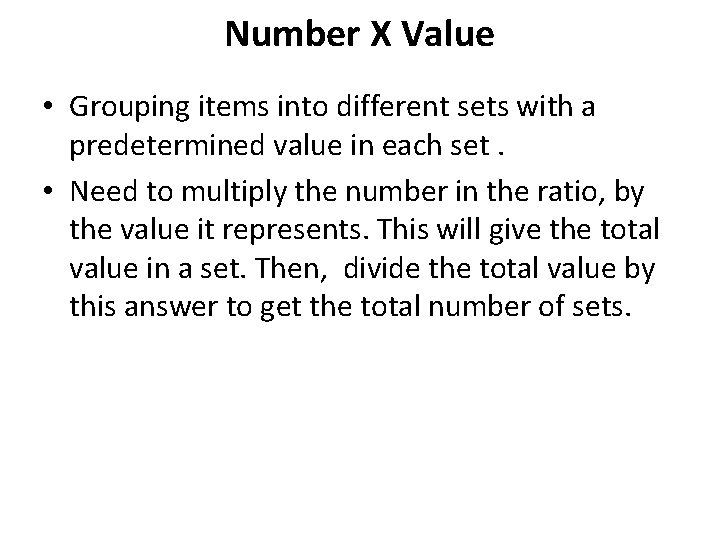Number X Value • Grouping items into different sets with a predetermined value in each set. • Need to multiply the number in the ratio, by the value it represents. This will give the total value in a set. Then, divide the total value by this answer to get the total number of sets.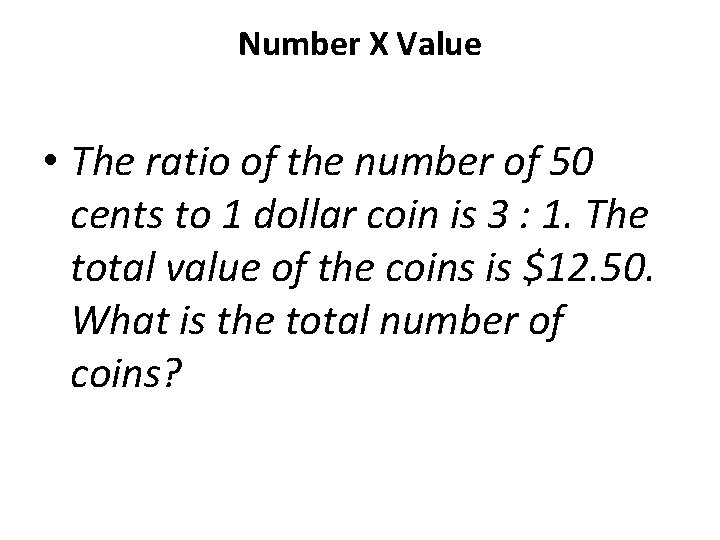Number X Value • The ratio of the number of 50 cents to 1 dollar coin is 3 : 1. The total value of the coins is \$12. 50. What is the total number of coins?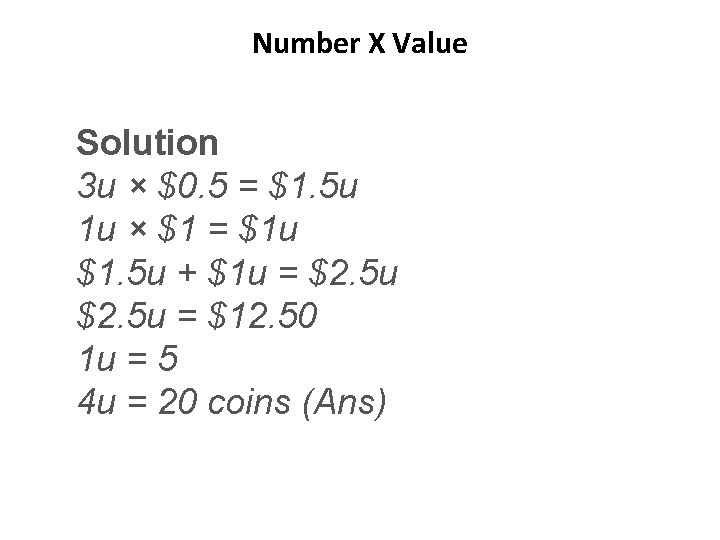Number X Value Solution 3 u × \$0. 5 = \$1. 5 u 1 u × \$1 = \$1 u \$1. 5 u + \$1 u = \$2. 5 u = \$12. 50 1 u = 5 4 u = 20 coins (Ans)